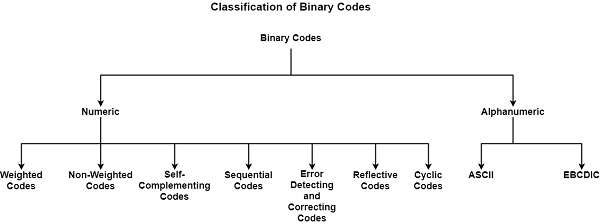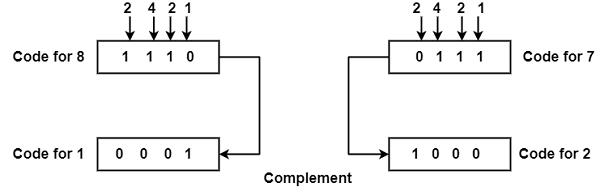# What are Binary Codes?

Digital data is described, saved, and communicated as sets of binary digits which are known as bits. The set of bits is called binary code. Binary codes are used in devices as they enable computers to implement complex evaluations fastly and effectively.

## Classification of Binary Codes

Binary codes can be defined as numbers and letters of the alphabet and some special characters and control services such as numeric or alphanumeric codes. Numeric codes can define numbers, whereas alphanumeric codes can define alphabetic letters and numerals. The binary codes are classified as demonstrated in the diagram.## Numeric Codes

Numeric codes can define numbers. There are the following numeric codes which are as follows −

• Weighted Binary Codes − Weighted binary codes are those codes that follow the positional weighting standards. In this code, each position of the number defines a particular weight. For example, in decimal code, if the number is 345, then the weight of 3 is 100, 4 is 10, and 5 is 1. In the 8421 weighted binary code, each digit has a weight of 8, 4, 2, or 1 equivalent to its position.

• Non-Weighted Binary Codes − These codes do not follow the positional weighting standards. In non-weighted codes, each digit position within the number does not have any permanent value.

Example − Excess-3 and Gray codes are examples of non-weighted codes. Excess-3 codes can represent decimal numbers. The code can be changed from the natural BCD (8421) code by inserting 3 (011 in binary) to the coded number. It is used in decimal arithmetic units. The excess-3 code of 1000 in 8421 is 1011.

Gray codes define each number in the series of integers {0...2^N1} as a binary string of length N including the adjacent integers have Gray code representations which distinct in only a one-bit position.

• Reflective Codes − A code is reflective when the code for 9 is a complement for the code for 0, 8 for 1, 7 for 2, 6 for 3, and 5 for 4.

Example − Codes 2421 and excess-3 are reflective. In code 2421: we understand that the positional weights are 2, 4, 2, and 1. The following diagram displays an example of reflective code where the complement of 8 is 1 and the complement of 7 is 2.• Sequential Codes − Sequential codes are codes in which the succeeding code is one binary number higher than its previous code. An example of sequential codes is 8421 and excess-3 codes.

• Cyclic Codes − In cyclic codes, only one bit in the code modifies at a time while changing from one number to the next. It is a non-weighted code, which defines that the position of the bit does not include any weight.

• Error Detecting Codes − When information is sent from one point to another, there is a probability that the information can get corrupted. To identify these data errors, there are various codes known as error detection codes.

• Error Correcting Codes − These codes not only identify errors in data but also accurately them necessarily. These are those approaches by which a collection of symbols can be defined such that even if any 1 bit of the description has unintentionally thrown, we can definitely recognize the previous symbol.

• Alphanumeric Codes − These are codes that include both numbers and alphabets. The most frequently used alphanumeric codes are ASCII and EBCDIC.

• EBCDIC Code − EBCDIC represents Extended Binary Coded Decimal Interchange. It is generally used with large computer systems such as mainframe computers. It is an 8-bit code that contains up to 256 characters.

• ASCII Code &minus ASCII stands for American Standard Code for Information Interchange. It has developed into a standard alphanumeric code for microcomputers and computers. It is a 7-bit code, which describes 128 various characters.